# CBSE Sample Papers for Class 12 Maths UnSolved 2016 Set 10

0
1066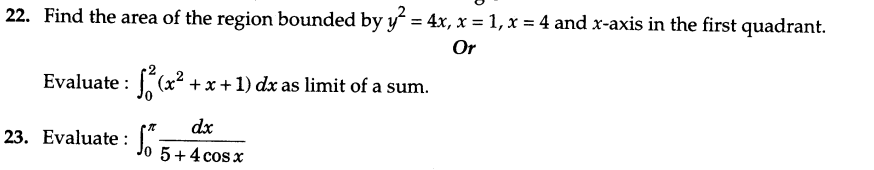SECTION —A

Question numbers l to 6 carry 1 mark each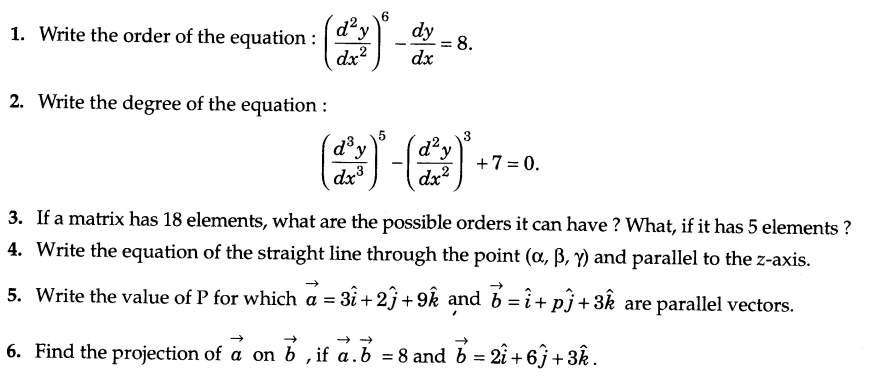SECTION — B

Question numbers 7 to 19 carry 4 marks each.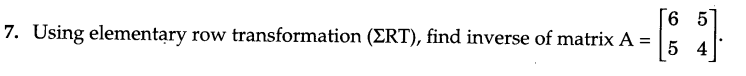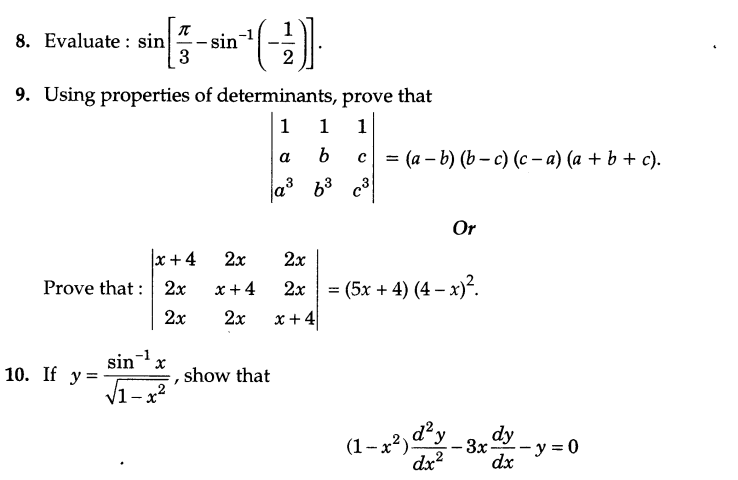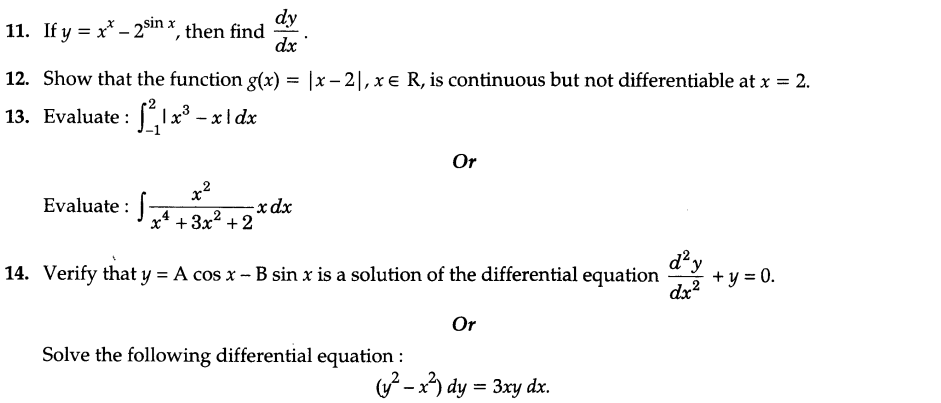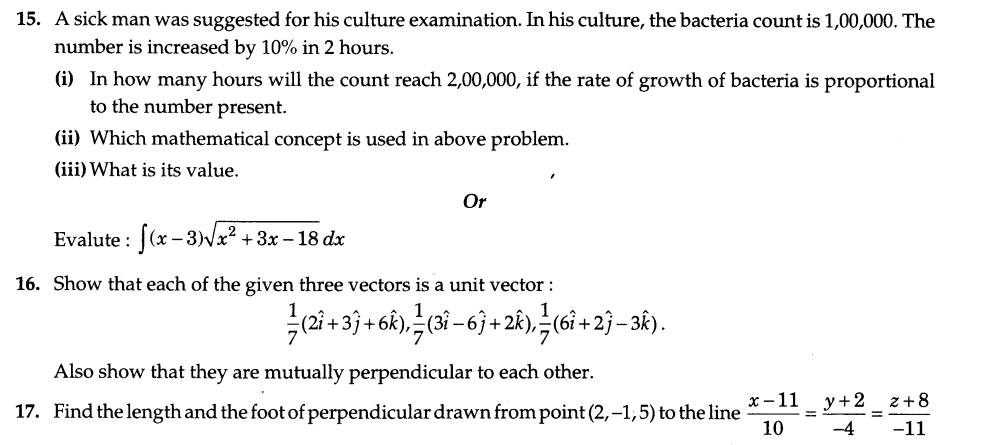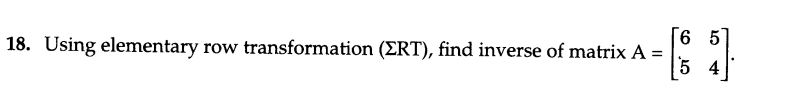19.A speaks truth 8 times out of 10 times. A dice is tossed. He reports that it was 5. What is the probability that it was actually 5 ?

SECTION — C

Question numbers 20 to 26 carry 6 marks each.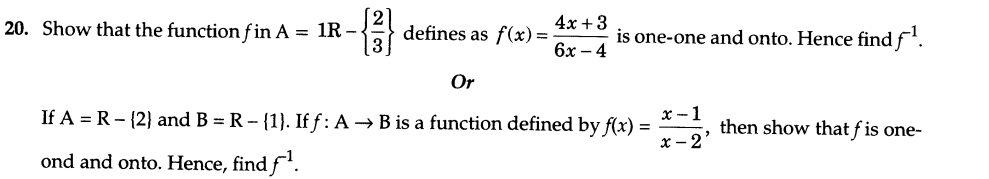21.Find the equation of the line through the point P(3, 4) which cuts from the first quadrant a triangle of the minimum area. Also, find the area of the triangle.24.Show that the line joining the origin to the point (2,1,1) is perpendicular to the line determined by the points (3,5, -1), (4, 3, -1).

25.There are two types of fertilizers Fj and F2. Fj contain 10% nitrogen and 6% phosphoric acid and F2consists of 5% of nitrogen and 10% phosphoric acid. After testing the soil conditions, a farmer finds that fie needs at least 14 kg of nitrogen and 14 kg of phosphoric acid for the crop. If F1 costs Rs 6/kg and F2costs Rs 5/kg, determine how much of each type of fertilizer should be used so that nutrient requirements are meet at a minimum cost. What is the minimum cost ? What is the value ?

26.An electronic assembly consists of two subsystems say A and B. From the previous testing procedures, the following probabilities are assumed to be known :
P(A fails) = 0.2, P(B fails alone) = 0.15, P(A and B fail) = 0.15.
Evaluate the following probabilities :
i)P(A fails/ B has failed)
ii)P(A fails alone).